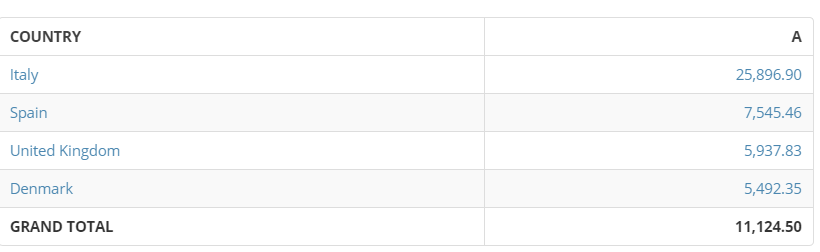# How can I total individual elements of a table?

Hi,

I have a card where I have individual country data calculated as such:The value of A is calculated simply as:

SUM(`X`)/SUM(`Y`)

The countries are in the same group, Europe.  However the Grand Total is simply completing the same "A" calculation across the group.  What I need is a simple sum of the indivdual country values; in this instance 25,896 + 7,545 + 5,937 + 5,492.  This is obviously simple in Excel and most likely the same in Tableau but appears to be a nightmare within Domo!

The data is originally in an Excel, howver I passed it into a Dataflow so as to share the calculations on the data set in an attempt to use a Sumo Card, however I get the same result.

Is there any advice that can be provided on how it can be possible to complete what should be such a simple calculation?

Thanks!